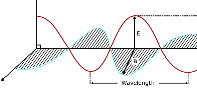# Ground (Surface) Wave## Ground or Surface Wave

A wave is said to be a ground wave or surface wave when it propagates from transmitter to receiver by gliding over the surface of the earth. This wave exists when

• both transmitting and receiving antennas are close to the earth and
• the antennas are vertically polarised.

Ground wave is useful at low-frequency broadcast applications. The broadcast signals received in the day-time are by ground waves. Ground wave signal is limited to only a few kilometers. The horizontally polarised antennas are not preferred as the horizontal component of the electric field in contact with the earth is short-circuited by the earth. When the wave is in contact with the earth, it induces charges in the earth and constitutes a current. The electric and magnetic fields of the wave over the earth are shown in figure below.

The earth behaves like a leaky capacitance in carrying the induced current. The equivalent circuit of the earth is shown in the figure below.

The characteristics of the earth are described by its fundamental constants namely permittivity, conductivity and permeability.

The wave is attenuated as it propagates due to the imperfect nature of the earth. The attenuation is mainly as a result of the absorption and reflection of EM energy by the earth. A part of this lost energy is compensated by the diffraction of additional energy coming from the atmospheric region above the earth.

## Ground Wave Field Strength

According to Somerfield analysis, the ground wave field strength for the flat earth is given by

$E=\frac{AE_{0}}{d}$

where

E = field strength at a point, V/m

E0 = field strength of the wave at a unit distance from the transmitting antenna, neglecting earth’s losses (V/m)

A = factor of the ground losses

d = distance of the point from Transmitting antenna

### E0 depends on

Power radiated by the transmitting antenna.

Directivity of the antenna in vertical and horizontal planes.

The factor, A depends on

Conductivity, σ mho/m

The permittivity of the earth, r

Frequency of the wave, f

Distance from the transmitter, d.

The determination of field strength due to Somerfield analysis mainly consists of the determination of A.

This is found from the knowledge of numerical distance, p and phase constant, b. For vertical polarisation, these are given by

$p=\frac{\pi }{D_{f}}\frac{d}{\lambda }cos\left ( b \right )$$b=tan^{-1}\left ( \frac{\epsilon _{r}+1}{D_{f}} \right )$

Where,

$D_{f}=1.80\times 10^{12}\frac{\sigma }{f}$

Df is known as dissipation factor of the dielectric

σ = conductivity, (mho)/cm of earth

r = relative permittivity of earth

f = frequency in Hz

d/λ = normalised distance with respect to λ

λ = wavelength

## The features of ground wave propagation

1. Ground wave propagates by gliding over the surface of the earth.
2. It exists for vertically polarised antennas.
3. These waves exist for antennas close to the earth.
4. It is suitable for VLF, LF and MF communications.
5. It can be used even at 15 kHz and up to 2 MHz.
6. Ground wave field strength is $E=\frac{AE_{0}}{d}$.
7. The ground wave field strength varies with the characteristics of the earth.
8. Ground waves require relatively high transmitter power.
9. Ground wave propagation losses vary considerably with the type of earth.
10. Thease waves are not affected by the changes in atmospheric conditions.
11. Ground waves can be used to communicate between any two points on the globe if there is sufficient transmitter power.
12. It can be used for radio navigation, ship-to-ship, ship-to-shore communication, and maritime mobile communications.